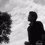# Sup and inf of constant function

Problem: Show that the constant function is integrable and find its value of integration.

Suppose $$f:\mathbb [a,b]\to \mathbb R$$ such that $$f(x)=\lambda$$ where $$\lambda$$ is any constant. Let $$P$$ be any partition on $$[a,b]$$, ie $P=\left\{a=t_0<t_1<t_2\cdots< t_n=b\right\}$ then Upper Darboux sum and Lower Darboux sum we evaluate by $U(f,P)=\sum_{1\leq k\leq n}\operatorname{Sup}\left\{f(x): x\in [t_{k-1},t_k]\right\}(t_k-t_{k-1})\\ L(f,P)=\sum_{1\leq k\leq n}\operatorname{inf}\left\{f(x): x\in [t_{k-1},t_k]\right\}(t_k-t_{k-1})$ Now what about the supremum and infimum of $f(x)$? If $\operatorname{sup}\left\{f(x): x\in[a,b]\right\}=\lambda$ but then $f(x)$ is constant so infimum of $f(x)$ is also $\lambda$ which immediately follows that $L(f,P)=\lambda(b-a)=U(f,P)$ Further $L(f)\geq L(f,P) ,\; U(f)\leq U(f,P) \implies L(f)=U(f)=\lambda(b-a)$ shows that $f(x)$ is integrable and its values is$L(f)\leq \int_a^b f(x) \leq U(f)\implies \int_a^b f(x) dx =\lambda(b-a)$

Now how to show that the supremum and infimum of the constant function is constant itself without using completeness property?

Any sorts of help will be appreciated.Note by Naren Bhandari
1 year ago

This discussion board is a place to discuss our Daily Challenges and the math and science related to those challenges. Explanations are more than just a solution — they should explain the steps and thinking strategies that you used to obtain the solution. Comments should further the discussion of math and science.

When posting on Brilliant:

• Use the emojis to react to an explanation, whether you're congratulating a job well done , or just really confused .
• Ask specific questions about the challenge or the steps in somebody's explanation. Well-posed questions can add a lot to the discussion, but posting "I don't understand!" doesn't help anyone.
• Try to contribute something new to the discussion, whether it is an extension, generalization or other idea related to the challenge.
• Stay on topic — we're all here to learn more about math and science, not to hear about your favorite get-rich-quick scheme or current world events.

MarkdownAppears as
*italics* or _italics_ italics
**bold** or __bold__ bold
- bulleted- list
• bulleted
• list
1. numbered2. list
1. numbered
2. list
Note: you must add a full line of space before and after lists for them to show up correctly
paragraph 1paragraph 2

paragraph 1

paragraph 2

[example link](https://brilliant.org)example link
> This is a quote
This is a quote
    # I indented these lines
# 4 spaces, and now they show
# up as a code block.

print "hello world"
# I indented these lines
# 4 spaces, and now they show
# up as a code block.

print "hello world"
MathAppears as
Remember to wrap math in $$ ... $$ or $ ... $ to ensure proper formatting.
2 \times 3 $2 \times 3$
2^{34} $2^{34}$
a_{i-1} $a_{i-1}$
\frac{2}{3} $\frac{2}{3}$
\sqrt{2} $\sqrt{2}$
\sum_{i=1}^3 $\sum_{i=1}^3$
\sin \theta $\sin \theta$
\boxed{123} $\boxed{123}$

## Comments

There are no comments in this discussion.

×

Problem Loading...

Note Loading...

Set Loading...